# Mean State

Period Mean (original grids) [W/m2]
Model Period Mean (intersection) [W/m2]
Model Period Mean (complement) [W/m2]
Benchmark Period Mean (intersection) [W/m2]
Benchmark Period Mean (complement) [W/m2]
Bias [W/m2]
RMSE [W/m2]
Phase Shift [months]
Bias Score 
RMSE Score 
Seasonal Cycle Score 
Spatial Distribution Score 
Interannual Variability Score 
Overall Score 
Benchmark [-] 131.
CRUNCEPv7 [-] 138. 139. 0.00 130. 157. 9.19 21.4 1.29 0.46 0.46 0.83 0.99 0.51 0.62
GSWP3v1 [-] 118. 119. 0.00 130. 157. -11.1 20.8 1.31 0.56 0.43 0.82 0.99 0.68 0.65
WFDEI [-] 124. 124. 0.00 130. 157. -4.96 21.8 1.65 0.61 0.36 0.77 0.99 0.71 0.63
Period Mean (original grids) [W/m2]
Model Period Mean (intersection) [W/m2]
Model Period Mean (complement) [W/m2]
Benchmark Period Mean (intersection) [W/m2]
Benchmark Period Mean (complement) [W/m2]
Bias [W/m2]
RMSE [W/m2]
Phase Shift [months]
Bias Score 
RMSE Score 
Seasonal Cycle Score 
Spatial Distribution Score 
Interannual Variability Score 
Overall Score 
Benchmark [-] 123.
CRUNCEPv7 [-] 114. 114. 0.00 122. 160. -7.78 23.8 1.58 0.59 0.47 0.75 0.97 0.58 0.64
GSWP3v1 [-] 103. 102. 0.00 122. 160. -19.8 25.9 1.34 0.46 0.51 0.80 0.99 0.63 0.65
WFDEI [-] 116. 115. 0.00 122. 160. -6.24 21.3 1.66 0.68 0.47 0.73 0.95 0.69 0.67
Period Mean (original grids) [W/m2]
Model Period Mean (intersection) [W/m2]
Model Period Mean (complement) [W/m2]
Benchmark Period Mean (intersection) [W/m2]
Benchmark Period Mean (complement) [W/m2]
Bias [W/m2]
RMSE [W/m2]
Phase Shift [months]
Bias Score 
RMSE Score 
Seasonal Cycle Score 
Spatial Distribution Score 
Interannual Variability Score 
Overall Score 
Benchmark [-] 123.
CRUNCEPv7 [-] 118. 118. 0.00 121. 157. -1.51 18.3 1.18 0.74 0.64 0.85 0.99 0.53 0.73
GSWP3v1 [-] 111. 111. 0.00 121. 157. -9.58 21.4 1.39 0.72 0.59 0.79 0.97 0.66 0.72
WFDEI [-] 120. 120. 0.00 121. 157. -0.912 19.6 1.46 0.78 0.59 0.79 0.96 0.66 0.73
Period Mean (original grids) [W/m2]
Model Period Mean (intersection) [W/m2]
Model Period Mean (complement) [W/m2]
Benchmark Period Mean (intersection) [W/m2]
Benchmark Period Mean (complement) [W/m2]
Bias [W/m2]
RMSE [W/m2]
Phase Shift [months]
Bias Score 
RMSE Score 
Seasonal Cycle Score 
Spatial Distribution Score 
Interannual Variability Score 
Overall Score 
Benchmark [-] 34.4
CRUNCEPv7 [-] 13.3 13.4 0.00 34.5 33.0 -22.2 28.0 0.368 0.68 0.74 0.98 0.86 0.67 0.78
GSWP3v1 [-] 30.4 30.5 0.00 34.5 33.0 -5.29 20.6 0.260 0.87 0.72 0.98 0.88 0.75 0.82
WFDEI [-] 5.05 5.15 0.00 34.5 33.0 -30.0 35.8 0.314 0.59 0.71 0.98 0.64 0.80 0.74
Period Mean (original grids) [W/m2]
Model Period Mean (intersection) [W/m2]
Model Period Mean (complement) [W/m2]
Benchmark Period Mean (intersection) [W/m2]
Benchmark Period Mean (complement) [W/m2]
Bias [W/m2]
RMSE [W/m2]
Phase Shift [months]
Bias Score 
RMSE Score 
Seasonal Cycle Score 
Spatial Distribution Score 
Interannual Variability Score 
Overall Score 
Benchmark [-] 147.
CRUNCEPv7 [-] 160. 160. 0.00 145. 156. 17.4 25.3 1.53 0.38 0.45 0.77 1.0 0.51 0.59
GSWP3v1 [-] 130. 130. 0.00 145. 156. -10.4 20.4 1.73 0.53 0.43 0.73 0.99 0.69 0.63
WFDEI [-] 144. 144. 0.00 145. 156. 2.30 20.8 2.10 0.56 0.40 0.68 0.97 0.64 0.61
Period Mean (original grids) [W/m2]
Model Period Mean (intersection) [W/m2]
Model Period Mean (complement) [W/m2]
Benchmark Period Mean (intersection) [W/m2]
Benchmark Period Mean (complement) [W/m2]
Bias [W/m2]
RMSE [W/m2]
Phase Shift [months]
Bias Score 
RMSE Score 
Seasonal Cycle Score 
Spatial Distribution Score 
Interannual Variability Score 
Overall Score 
Benchmark [-] 53.7
CRUNCEPv7 [-] 43.1 43.1 0.00 53.5 64.9 -10.3 22.3 0.375 0.83 0.72 0.97 0.85 0.77 0.81
GSWP3v1 [-] 50.1 50.1 0.00 53.5 64.9 -3.17 16.2 0.216 0.90 0.78 0.99 0.91 0.70 0.84
WFDEI [-] 31.8 31.8 0.00 53.5 64.9 -21.5 28.0 0.216 0.70 0.73 0.99 0.78 0.84 0.79
Period Mean (original grids) [W/m2]
Model Period Mean (intersection) [W/m2]
Model Period Mean (complement) [W/m2]
Benchmark Period Mean (intersection) [W/m2]
Benchmark Period Mean (complement) [W/m2]
Bias [W/m2]
RMSE [W/m2]
Phase Shift [months]
Bias Score 
RMSE Score 
Seasonal Cycle Score 
Spatial Distribution Score 
Interannual Variability Score 
Overall Score 
Benchmark [-] 71.7
CRUNCEPv7 [-] 66.4 66.1 0.00 71.1 90.6 -3.70 21.5 0.523 0.79 0.69 0.95 0.94 0.71 0.80
GSWP3v1 [-] 62.4 62.3 0.00 71.1 90.6 -7.46 18.8 0.447 0.81 0.72 0.96 0.99 0.68 0.81
WFDEI [-] 62.4 62.1 0.00 71.1 90.6 -7.84 22.3 0.530 0.80 0.67 0.95 0.94 0.75 0.80
Period Mean (original grids) [W/m2]
Model Period Mean (intersection) [W/m2]
Model Period Mean (complement) [W/m2]
Benchmark Period Mean (intersection) [W/m2]
Benchmark Period Mean (complement) [W/m2]
Bias [W/m2]
RMSE [W/m2]
Phase Shift [months]
Bias Score 
RMSE Score 
Seasonal Cycle Score 
Spatial Distribution Score 
Interannual Variability Score 
Overall Score 
Benchmark [-] 120.
CRUNCEPv7 [-] 125. 126. 0.00 118. 153. 11.6 25.2 0.984 0.58 0.57 0.87 0.95 0.62 0.69
GSWP3v1 [-] 98.3 98.0 0.00 118. 153. -15.7 25.1 0.690 0.60 0.55 0.92 0.99 0.65 0.71
WFDEI [-] 114. 114. 0.00 118. 153. -0.0828 22.5 0.772 0.73 0.53 0.91 1.0 0.67 0.73
Period Mean (original grids) [W/m2]
Model Period Mean (intersection) [W/m2]
Model Period Mean (complement) [W/m2]
Benchmark Period Mean (intersection) [W/m2]
Benchmark Period Mean (complement) [W/m2]
Bias [W/m2]
RMSE [W/m2]
Phase Shift [months]
Bias Score 
RMSE Score 
Seasonal Cycle Score 
Spatial Distribution Score 
Interannual Variability Score 
Overall Score 
Benchmark [-] 30.5
CRUNCEPv7 [-] 7.68 8.06 0.00 31.0 19.5 -24.1 29.5 0.351 0.64 0.73 0.98 0.82 0.73 0.77
GSWP3v1 [-] 21.5 21.8 0.00 31.0 19.5 -10.1 20.4 0.190 0.83 0.73 0.99 0.98 0.76 0.84
WFDEI [-] -3.46 -3.10 0.00 31.0 19.5 -34.9 39.8 0.351 0.53 0.70 0.98 0.43 0.83 0.69
Period Mean (original grids) [W/m2]
Model Period Mean (intersection) [W/m2]
Model Period Mean (complement) [W/m2]
Benchmark Period Mean (intersection) [W/m2]
Benchmark Period Mean (complement) [W/m2]
Bias [W/m2]
RMSE [W/m2]
Phase Shift [months]
Bias Score 
RMSE Score 
Seasonal Cycle Score 
Spatial Distribution Score 
Interannual Variability Score 
Overall Score 
Benchmark [-] 109.
CRUNCEPv7 [-] 101. 101. 0.00 108. 131. -5.52 16.4 0.661 0.84 0.74 0.95 0.99 0.64 0.82
GSWP3v1 [-] 95.3 95.3 0.00 108. 131. -10.6 18.6 0.509 0.78 0.74 0.96 0.98 0.72 0.82
WFDEI [-] 102. 102. 0.00 108. 131. -5.32 17.9 0.555 0.85 0.71 0.95 1.0 0.75 0.83
Period Mean (original grids) [W/m2]
Model Period Mean (intersection) [W/m2]
Model Period Mean (complement) [W/m2]
Benchmark Period Mean (intersection) [W/m2]
Benchmark Period Mean (complement) [W/m2]
Bias [W/m2]
RMSE [W/m2]
Phase Shift [months]
Bias Score 
RMSE Score 
Seasonal Cycle Score 
Spatial Distribution Score 
Interannual Variability Score 
Overall Score 
Benchmark [-] 65.7
CRUNCEPv7 [-] 62.7 62.8 0.00 63.8 85.4 -0.347 22.2 0.341 0.85 0.72 0.98 0.95 0.79 0.83
GSWP3v1 [-] 55.9 55.9 0.00 63.8 85.4 -5.87 16.7 0.233 0.89 0.78 0.98 0.99 0.66 0.85
WFDEI [-] 46.7 46.7 0.00 63.8 85.4 -15.0 24.8 0.347 0.78 0.73 0.98 0.91 0.82 0.82
Period Mean (original grids) [W/m2]
Model Period Mean (intersection) [W/m2]
Model Period Mean (complement) [W/m2]
Benchmark Period Mean (intersection) [W/m2]
Benchmark Period Mean (complement) [W/m2]
Bias [W/m2]
RMSE [W/m2]
Phase Shift [months]
Bias Score 
RMSE Score 
Seasonal Cycle Score 
Spatial Distribution Score 
Interannual Variability Score 
Overall Score 
Benchmark [-] 109.
CRUNCEPv7 [-] 73.1 73.1 0.00 80.5 123. -5.80 22.4 0.719 0.66 0.62 0.92 0.96 0.60 0.73
GSWP3v1 [-] 66.4 66.4 0.00 80.5 123. -12.5 22.0 0.682 0.66 0.62 0.92 0.98 0.67 0.74
WFDEI [-] 68.0 68.0 0.00 80.5 123. -11.2 23.9 0.782 0.68 0.60 0.90 0.96 0.71 0.74
Period Mean (original grids) [W/m2]
Model Period Mean (intersection) [W/m2]
Model Period Mean (complement) [W/m2]
Benchmark Period Mean (intersection) [W/m2]
Benchmark Period Mean (complement) [W/m2]
Bias [W/m2]
RMSE [W/m2]
Phase Shift [months]
Bias Score 
RMSE Score 
Seasonal Cycle Score 
Spatial Distribution Score 
Interannual Variability Score 
Overall Score 
Benchmark [-] 76.6
CRUNCEPv7 [-] 64.5 64.6 0.00 73.9 144. -7.02 19.6 0.915 0.68 0.62 0.90 0.96 0.61 0.73
GSWP3v1 [-] 53.5 53.5 0.00 73.9 144. -18.1 24.5 0.894 0.55 0.62 0.90 0.94 0.70 0.72
WFDEI [-] 64.6 64.7 0.00 73.9 144. -7.27 18.7 0.912 0.72 0.63 0.89 0.92 0.63 0.73
Period Mean (original grids) [W/m2]
Model Period Mean (intersection) [W/m2]
Model Period Mean (complement) [W/m2]
Benchmark Period Mean (intersection) [W/m2]
Benchmark Period Mean (complement) [W/m2]
Bias [W/m2]
RMSE [W/m2]
Phase Shift [months]
Bias Score 
RMSE Score 
Seasonal Cycle Score 
Spatial Distribution Score 
Interannual Variability Score 
Overall Score 
Benchmark [-] 36.2
CRUNCEPv7 [-] 13.9 13.7 0.00 36.2 36.3 -24.2 30.5 0.113 0.65 0.71 0.99 0.82 0.60 0.75
GSWP3v1 [-] 25.8 25.6 0.00 36.2 36.3 -12.3 21.9 0.0864 0.80 0.73 0.99 0.95 0.78 0.83
WFDEI [-] 4.52 4.43 0.00 36.2 36.3 -33.1 38.9 0.133 0.55 0.69 0.99 0.63 0.76 0.72
Period Mean (original grids) [W/m2]
Model Period Mean (intersection) [W/m2]
Model Period Mean (complement) [W/m2]
Benchmark Period Mean (intersection) [W/m2]
Benchmark Period Mean (complement) [W/m2]
Bias [W/m2]
RMSE [W/m2]
Phase Shift [months]
Bias Score 
RMSE Score 
Seasonal Cycle Score 
Spatial Distribution Score 
Interannual Variability Score 
Overall Score 
Benchmark [-] 138.
CRUNCEPv7 [-] 120. 120. 0.00 133. 161. -4.91 26.0 1.10 0.56 0.64 0.87 0.82 0.66 0.70
GSWP3v1 [-] 110. 111. 0.00 133. 161. -16.9 25.1 1.14 0.60 0.63 0.85 0.99 0.68 0.73
WFDEI [-] 124. 125. 0.00 133. 161. -3.68 20.1 1.31 0.73 0.61 0.82 0.99 0.69 0.74
Period Mean (original grids) [W/m2]
Model Period Mean (intersection) [W/m2]
Model Period Mean (complement) [W/m2]
Benchmark Period Mean (intersection) [W/m2]
Benchmark Period Mean (complement) [W/m2]
Bias [W/m2]
RMSE [W/m2]
Phase Shift [months]
Bias Score 
RMSE Score 
Seasonal Cycle Score 
Spatial Distribution Score 
Interannual Variability Score 
Overall Score 
Benchmark [-] 98.6
CRUNCEPv7 [-] 89.2 89.5 0.00 96.9 135. -5.58 19.5 0.374 0.86 0.73 0.97 0.96 0.63 0.81
GSWP3v1 [-] 83.4 83.5 0.00 96.9 135. -11.8 18.1 0.427 0.80 0.78 0.97 0.97 0.72 0.84
WFDEI [-] 85.0 85.1 0.00 96.9 135. -9.84 23.1 0.380 0.83 0.68 0.97 0.97 0.84 0.83
Period Mean (original grids) [W/m2]
Model Period Mean (intersection) [W/m2]
Model Period Mean (complement) [W/m2]
Benchmark Period Mean (intersection) [W/m2]
Benchmark Period Mean (complement) [W/m2]
Bias [W/m2]
RMSE [W/m2]
Phase Shift [months]
Bias Score 
RMSE Score 
Seasonal Cycle Score 
Spatial Distribution Score 
Interannual Variability Score 
Overall Score 
Benchmark [-] 63.9
CRUNCEPv7 [-] 53.5 53.6 0.00 63.6 72.9 -10.0 22.7 0.351 0.84 0.71 0.98 0.94 0.59 0.80
GSWP3v1 [-] 57.5 57.6 0.00 63.6 72.9 -5.89 15.1 0.143 0.89 0.80 0.99 0.99 0.70 0.86
WFDEI [-] 44.7 44.8 0.00 63.6 72.9 -18.8 27.1 0.252 0.73 0.72 0.98 0.95 0.86 0.83
Period Mean (original grids) [W/m2]
Model Period Mean (intersection) [W/m2]
Model Period Mean (complement) [W/m2]
Benchmark Period Mean (intersection) [W/m2]
Benchmark Period Mean (complement) [W/m2]
Bias [W/m2]
RMSE [W/m2]
Phase Shift [months]
Bias Score 
RMSE Score 
Seasonal Cycle Score 
Spatial Distribution Score 
Interannual Variability Score 
Overall Score 
Benchmark [-] 82.3
CRUNCEPv7 [-] 65.2 65.7 0.00 81.8 110. -15.5 19.8 0.373 0.75 0.79 0.97 0.99 0.62 0.82
GSWP3v1 [-] 73.4 73.6 0.00 81.8 110. -7.75 17.6 0.537 0.83 0.77 0.95 0.98 0.71 0.84
WFDEI [-] 79.4 79.7 0.00 81.8 110. -1.53 19.7 0.458 0.87 0.71 0.96 1.0 0.80 0.84
Period Mean (original grids) [W/m2]
Model Period Mean (intersection) [W/m2]
Model Period Mean (complement) [W/m2]
Benchmark Period Mean (intersection) [W/m2]
Benchmark Period Mean (complement) [W/m2]
Bias [W/m2]
RMSE [W/m2]
Phase Shift [months]
Bias Score 
RMSE Score 
Seasonal Cycle Score 
Spatial Distribution Score 
Interannual Variability Score 
Overall Score 
Benchmark [-] 139.
CRUNCEPv7 [-] 153. 153. 0.00 138. 170. 15.2 21.6 1.17 0.39 0.45 0.83 0.97 0.58 0.61
GSWP3v1 [-] 123. 123. 0.00 138. 170. -14.3 22.9 1.18 0.39 0.40 0.83 0.97 0.72 0.62
WFDEI [-] 135. 135. 0.00 138. 170. -3.13 20.3 1.49 0.62 0.35 0.79 0.99 0.68 0.63
Period Mean (original grids) [W/m2]
Model Period Mean (intersection) [W/m2]
Model Period Mean (complement) [W/m2]
Benchmark Period Mean (intersection) [W/m2]
Benchmark Period Mean (complement) [W/m2]
Bias [W/m2]
RMSE [W/m2]
Phase Shift [months]
Bias Score 
RMSE Score 
Seasonal Cycle Score 
Spatial Distribution Score 
Interannual Variability Score 
Overall Score 
Benchmark [-] 121.
CRUNCEPv7 [-] 125. 125. 0.00 120. 143. 5.47 17.3 0.799 0.78 0.66 0.92 0.99 0.59 0.77
GSWP3v1 [-] 110. 110. 0.00 120. 143. -9.58 20.2 1.04 0.72 0.62 0.87 0.89 0.67 0.73
WFDEI [-] 117. 117. 0.00 120. 143. -2.75 19.1 1.43 0.81 0.59 0.80 1.0 0.78 0.76
Period Mean (original grids) [W/m2]
Model Period Mean (intersection) [W/m2]
Model Period Mean (complement) [W/m2]
Benchmark Period Mean (intersection) [W/m2]
Benchmark Period Mean (complement) [W/m2]
Bias [W/m2]
RMSE [W/m2]
Phase Shift [months]
Bias Score 
RMSE Score 
Seasonal Cycle Score 
Spatial Distribution Score 
Interannual Variability Score 
Overall Score 
Benchmark [-] 98.1
CRUNCEPv7 [-] 84.7 84.2 0.00 95.8 118. -11.7 26.0 0.603 0.72 0.69 0.94 0.93 0.63 0.77
GSWP3v1 [-] 90.8 90.2 0.00 95.8 118. -6.74 23.5 0.495 0.76 0.71 0.96 0.99 0.63 0.79
WFDEI [-] 89.9 89.4 0.00 95.8 118. -8.16 25.8 0.712 0.73 0.69 0.92 0.97 0.67 0.78

# Temporally integrated period mean

BENCHMARK MEAN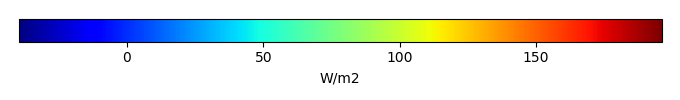MODEL MEANBIAS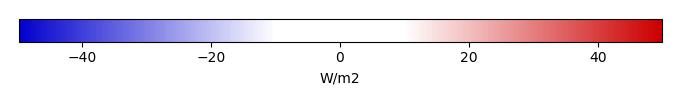BIAS SCORERMSE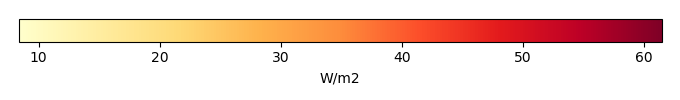RMSE SCOREBENCHMARK INTERANNUAL VARIABILITY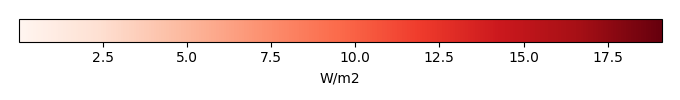MODEL INTERANNUAL VARIABILITYINTERANNUAL VARIABILITY SCOREBENCHMARK MAX MONTHMODEL MAX MONTHDIFFERENCE IN MAX MONTH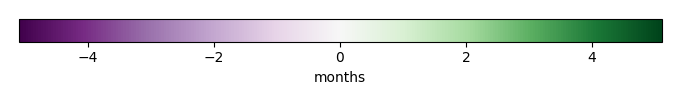SEASONAL CYCLE SCORESPATIAL TAYLOR DIAGRAMMODEL COLORS# Spatially integrated regional mean

MODEL COLORSREGIONAL MEANANNUAL CYCLEMONTHLY ANOMALYANNUAL CYCLE# All Models

BenchmarkCRUNCEPv7GSWP3v1WFDEI# Data Information

creation_date: Thu May 8 22:58:12 PDT 2014

source_file: This product is generated from monthly 1 degree CERES EBAF Radiation observations